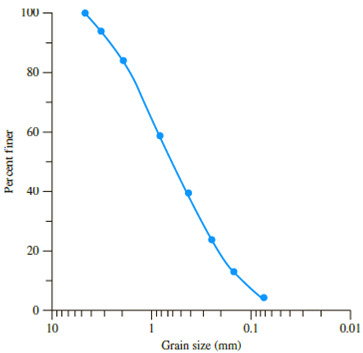Chapter 6, Problem 6.17PPrinciples of Geotechnical Enginee...

9th Edition
Braja M. Das + 1 other
ISBN: 9781305970939

Solutions

Chapter
SectionPrinciples of Geotechnical Enginee...

9th Edition
Braja M. Das + 1 other
ISBN: 9781305970939
Textbook Problem

Vibroflotation is being considered for in situ densification of a thick deposit of granular soils at a particular site. The results of the sieve analysis of the proposed backfill material is shown in Figure 6.41.Figure 6.41Determine the suitability number, SN, and rate it as a backfill material.

To determine

Find the suitability number and the rating for backfill material.

Explanation

Determine the suitability number SN using the relation.

SN=1.73(D50)2+1(D20)2+1(D50)2

Here, D50 is the diameter through the 50 % material passes, D20 is the diameter through the 20 % material passes, and D10 is the diameter through the 10 % material passes.

Refer Figure 6.41, take the D50 value as 0.6 mm, D20 value as 0.2 mm, and D10 value as 0

Still sussing out bartleby?

Check out a sample textbook solution.

See a sample solution

The Solution to Your Study Problems

Bartleby provides explanations to thousands of textbook problems written by our experts, many with advanced degrees!

Get Started

Describe the basic ideas of probability.

Engineering Fundamentals: An Introduction to Engineering (MindTap Course List)

What are two reasons for center drilling on the lathe?

Precision Machining Technology (MindTap Course List)

How would you balance DFDs?

Systems Analysis and Design (Shelly Cashman Series) (MindTap Course List)

What are the advantages of GMA welding?

Welding: Principles and Applications (MindTap Course List)